# 2s Complement Arithmetic

2’s complement has an interesting property that the binary arithmetic operation can be performed easily on signed or unsigned numbers. It yields correct 2’s complement in result.

### How to represent decimal number in 2’s complement?

Let us take a number. The negative signed that number is such number sum of which with this number is zero. Take examples of our most familiar number system that is decimal system.
Where,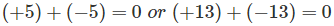In binary system there are only two digits 0 and 1. In binary system each and every number and its sign are represented by using only these two digits 0 and 1. As the negative and positive signs can not be written directly in binary system, we have to find some alternative method of representing them.

For that we take advantage of definition of negative number. If we have to represent a negative binary number, we have to find a binary number sum of which with the original unsigned or positive binary number is zero. As the sum of these two numbers is zero, the second number is taken as negative equivalent of the first number.
Suppose we have to write – 5 in binary which is negative of 5 or + 5. Now 5 or + 5 can be represented in binary byte or 8 bits system as 0000 0101 and zero is represented in binary byte or 8 bits system as 0000 0000.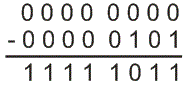Hence this result is representation of (- 5)10.

Now let us examine 2’s complement of 0000 0101 and this is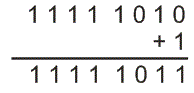Hence, it is proved zero minus any binary number is nothing but 2’s complement of this number, hence 2’s complement of any binary number can be represented as negative equivalent of that number. In 2’s complement first we convert a decimal number into binary number system. Suppose we want to represent (5)10 into binary 2’s complement. Binary representation of (5)10 is (0000 0101)2.

Now the interesting fact is that the unsigned form (5)10 into 2’s complement is direct representation of binary form of (5)10 i.e. (0000 0101)2. Unsigned number is treated as positive number. Signed decimal number can be a positive number or negative. Now to calculate 2’s complement for a signed decimal number i.e. (-5)10, we have to find out the 1’s complement and then convert each digit into its opposite digit (i.e. 1 into 0 or 0 into 1) and add 1 at last. This result after addition is the 2’s complete representation of signed (negative) decimal number.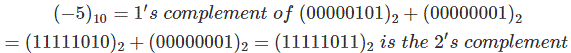Now look at the chart below.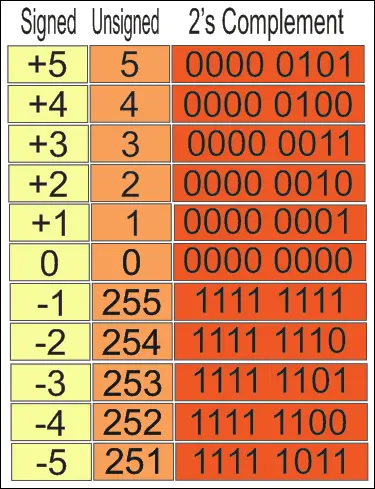(+5)10 is same as (5)10. But for (-1)10 it is treated as unsigned (255)10. (-5)10 ⇔ (251)10
Always keep it in the mind that negative 2’s complement numbers are always represented as the binary number in such a way that when added to a positive number of the same magnitude must be zero.
The important thing is to be kept in the mind that the left most bit or significant bit indicates the sign of the integer. It is called the signed bit also.

• If the left most bit is zero then the number is positive.
• If the left most bit is one then the number is negative.

## Arithmetic in 2’s Complement Method

Addition in the 2’s complement, it always follows the same rule as it is used in the normally binary addition. Suppose we want to add (8)10 and (-3)10.
First we have to convert them into 2’s complement and simply add them.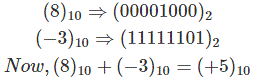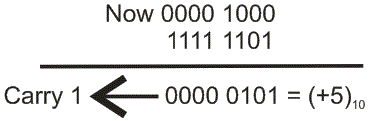As carry is 1 then number is positive. Here carry is 1 and it is out of 8 bits hence is not considered in byte and hence the answer is one byte long positive number. So it is clear that for signed and unsigned numbers addition can be performed easily in 2’s complement.

### 2’s Complement Subtraction

Subtraction in 2’s complement follows the same rule as it is in the normally binary addition. Only thing is that here subtrahend is first converted to its negative form and then it is added with minuend. Suppose we want to subtract (8)10 from (9)10. First convert (8)10 from (-9)10 into 2’s complement and simply add.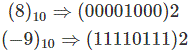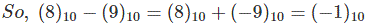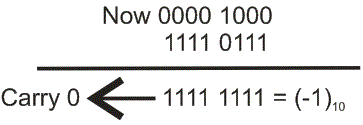As carry is 0 then number is negative. So it is clear that subtraction can be performed easily in 2’s complement easily.

### 2’s Complement Multiplication

For 2’s complement multiplication, it always follows the same rules as binary multiplication. Suppose we want to multiply (- 4)10 with (4)10 that gives (-16)10. Now , (- 4)10 = 1111 1100 in 2’s complement and (4)10 = 0000 0100 in 2’s complement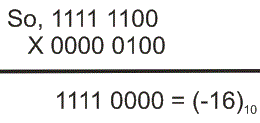### 2’s Complement Division

For the 2’s complement division, the method is 2’s complement subtraction repeatedly. First calculate the 2’s complement of the divisor and then this converted divisor is to be added to the dividend. Now come to the next subtraction cycle. Here quotient replaces the dividend. Repeats it again and again up to the quotient is getting too small or zero. If it is not zero then it is treated as remainder. Suppose we want to calculate (7)10 ÷ (3)10, answer gives (2)10 as quotient and (1)10 as remainder. Now let’s start to calculate it in 2’s complement.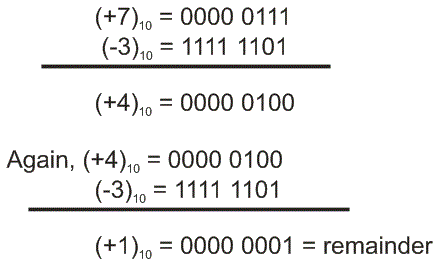As the repetition is in two rounds, then the quotient is (2)10.

Want To Learn Faster? 🎓
Get electrical articles delivered to your inbox every week.
No credit card required—it’s 100% free.